# Complex NumbersPage 1

#### WATCH ALL SLIDES

Slide 1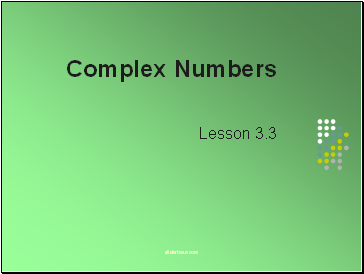Complex Numbers

Lesson 3.3

Slide 2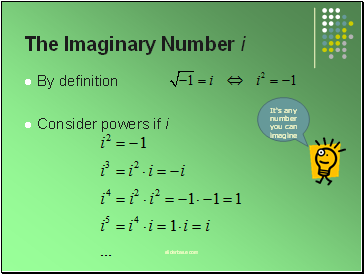## The Imaginary Number

By definition

Consider powers if i

Slide 3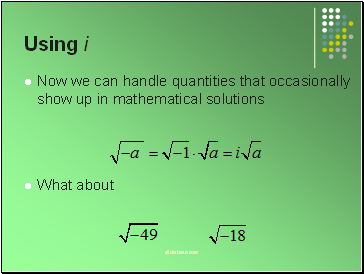## Using

Now we can handle quantities that occasionally show up in mathematical solutions

Slide 4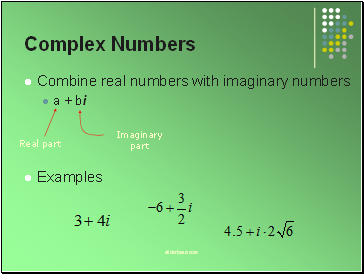## Complex Numbers

Combine real numbers with imaginary numbers

a + bi

Examples

Slide 5## Try It Out

Write these complex numbers in standard form a + bi

Slide 6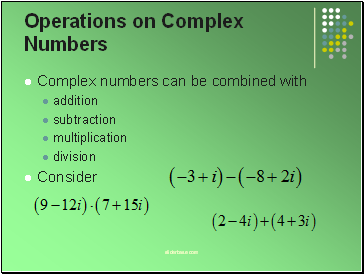## Operations on Complex Numbers

Complex numbers can be combined with

subtraction

multiplication

division

Consider

Slide 7Operations on Complex Numbers

Division technique

Multiply numerator and denominator by the conjugate of the denominator

Slide 8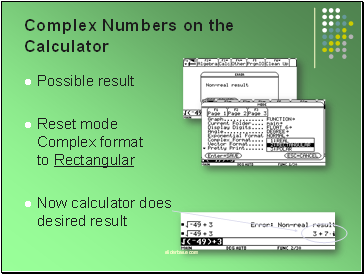## Complex Numbers on the Calculator

Possible result

Reset mode Complex format to Rectangular

Now calculator does desired result

Slide 9Complex Numbers on the Calculator

Operations with complex on calculator

Make sure to use the correct character for i. Use 2nd-i

Slide 10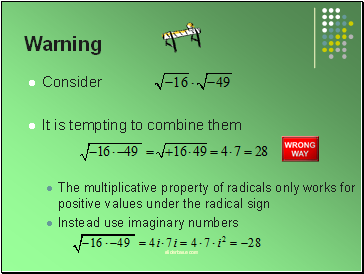## Warning

Consider

It is tempting to combine them

The multiplicative property of radicals only works for positive values under the radical sign

Slide 11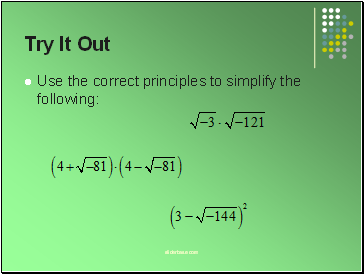Try It Out

Use the correct principles to simplify the following:

Slide 12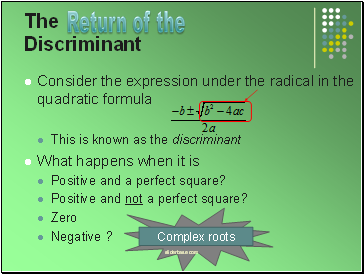## The Discriminant

This is known as the discriminant

What happens when it is

Positive and a perfect square?

Positive and not a perfect square?

Zero

Negative ?

Return of the

Slide 13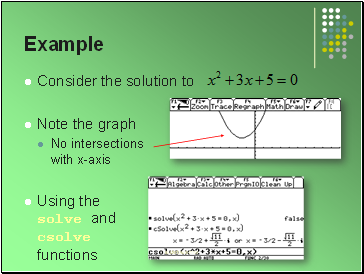## Example

Consider the solution to

Note the graph

No intersections with x-axis

Using the solve and csolve functions

Slide 14

Go to page:
1  2